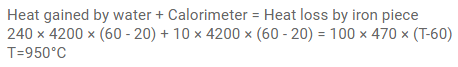# A piece of iron of mass 100 g is kept insideQuestion:

A piece of iron of mass $100 \mathrm{~g}$ is kept inside a furnace for a long time and then put in a calorimeter of water equivalent $10 \mathrm{~g}$ containing $240 \mathrm{~g}$ of water at $20^{\circ} \mathrm{C}$. The mixture attains an equilibrium temperature of $60^{\circ} \mathrm{C}$. Find the temperature of the furnace. Specific heat capacity of iron $=470 \mathrm{~J} / \mathrm{kg}-{ }^{\circ} \mathrm{C}$.

Solution: